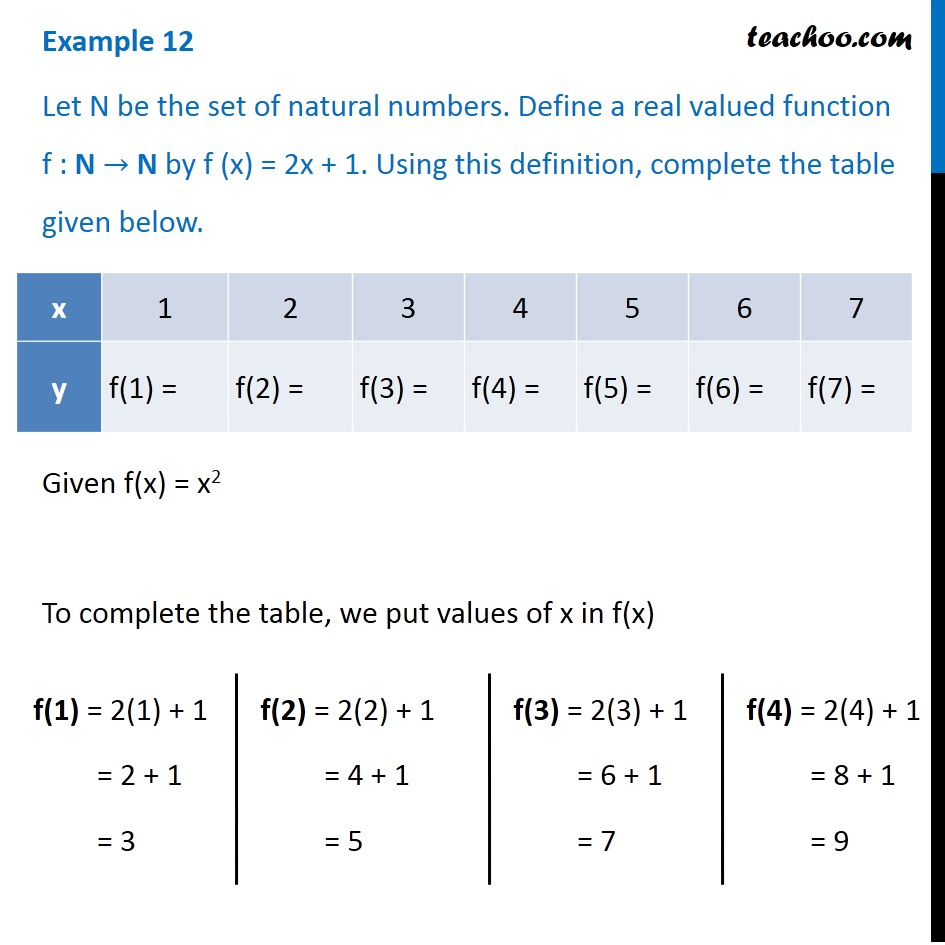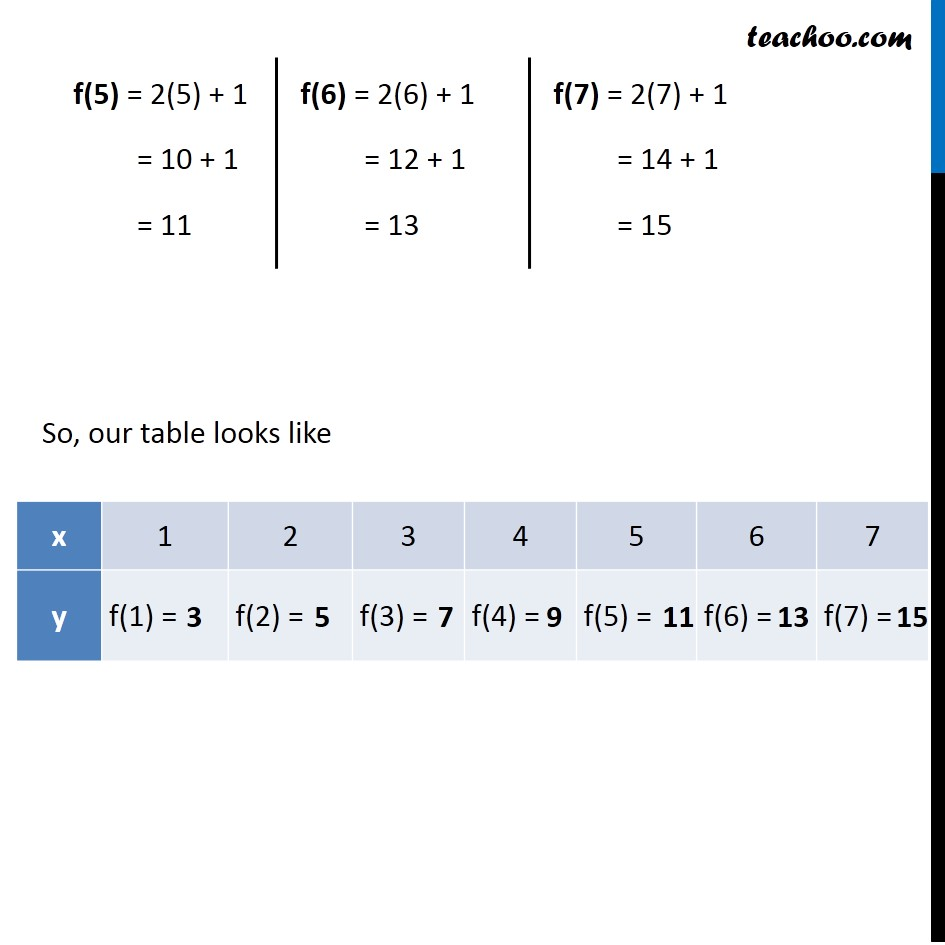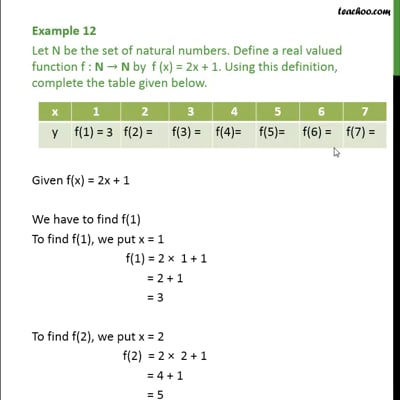Examples

Chapter 2 Class 11 Relations and Functions (Term 1)
Serial order wiseThis video is only available for Teachoo black users

### Transcript

Example 12 Let N be the set of natural numbers. Define a real valued function f : N → N by f (x) = 2x + 1. Using this definition, complete the table given below. Given f(x) = x2 To complete the table, we put values of x in f(x) f(1) = 2(1) + 1 = 2 + 1 = 3 f(2) = 2(2) + 1 = 4 + 1 = 5 f(3) = 2(3) + 1 = 6 + 1 = 7 f(4) = 2(4) + 1 = 8 + 1 = 9 f(5) = 2(5) + 1 = 10 + 1 = 11 f(6) = 2(6) + 1 = 12 + 1 = 13 f(7) = 2(7) + 1 = 14 + 1 = 15 So, our table looks like